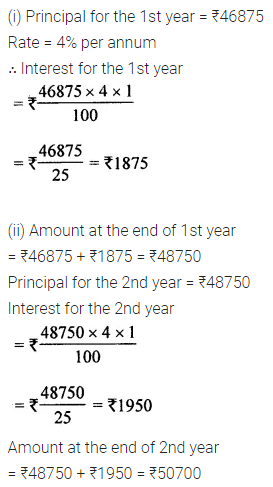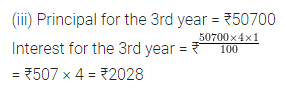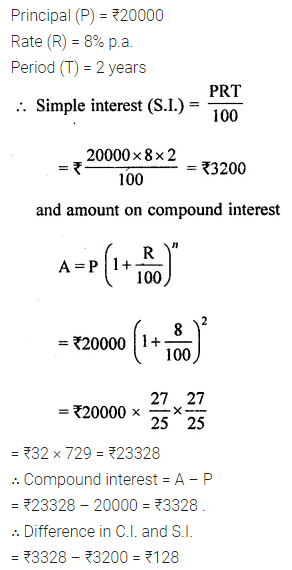# ML Aggarwal Class 8 Solutions for ICSE Maths Chapter 8 Simple and Compound Interest Ex 8.2

## ML Aggarwal Class 8 Solutions for ICSE Maths Chapter 8 Simple and Compound Interest Ex 8.2

Question 1.
Calculate the compound interest on ₹6000 at 10% per annum for two years.
Solution:Question 2.
Salma borrowed from Mahila Samiti a sum of ₹ 1875 to purchase a sewing machine. If the rate of interest is 4% per annum, what is the compound interest that she has to pay after 2 years?
Solution:Question 3.
Jacob invests ₹12000 for 3 years at 10% per annum. Calculate the amount and the compound interest that Jacob will get after 3 years.
Solution:Question 4.
A man invests ₹46875 at 4% per annum compound interest for 3 years.
Calculate:
(i) the interest for the first year.
(ii) the amount standing to his credit at the end of second year.
(iii) the interest for the third year.
Solution:Question 5.
Calculate the compound interest for the second year on ₹6000 invested for 3 years at 10% p.a. Also find the sum due at the end of third year.
Solution:Question 6.
Calculate the amount and the compound interest on ₹5000 in 2 years when the rate of interest for successive years is 6% and 8% respectively.
Solution:Question 7.
Calculate the difference between the compound interest and the simple interest on ₹20000 in 2 years at 8% per annum.
Solution: